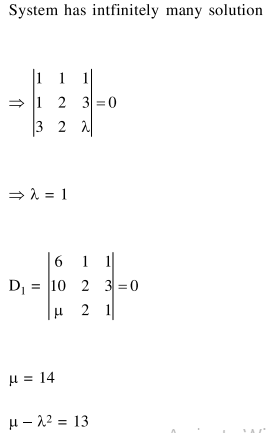# If the system of linear equations,`
Question:

If the system of linear equations,

$x+y+z=6$

$x+2 y+3 z=10$

$3 x+2 y+\lambda z=\mu$

has more two solutions, then $\mu-\lambda^{2}$ is equal to_________.

Solution: